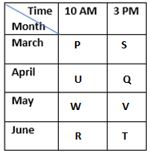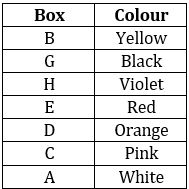Latest Banking jobs   »

# Reasoning Ability Quiz For IBPS Clerk/PO Prelims 2022- 6th September

Directions (1-5): Study the following information carefully and answer the given questions:
Eight students P, Q, R, S, T, U, V and W, appear in an examination in two different shifts either at 10 AM or 3 PM. They give exam on different months of the same year starting from March to June.
Only two persons give exam in between W and T, whose exam is in the month which have even number of days. P gives exam in the month having 31 days. S gives exam in evening shift but before W. Three persons give exam between U and R. W gives exam before T. V gives an exam in evening shift of the month which have odd number of days but after W. U gives the exam in the month having 30 days but not in June. Only two persons give exam between P and Q.

Q1. How many persons give exam in between Q and R?
(a) Three
(b) None
(c) Two
(d) One
(e) More than three

Q2. Who gives the exam at 10 AM in March?
(a) P
(b) U
(c) R
(d) T
(e) S

Q3. When did S appear for his exams?
(a) June- 10 AM
(b) March- 3 PM
(c) June- 3 PM
(d) April- 3 PM
(e) May- 10 AM

Q4. Which of the following combination is correct?
(a) V- April- 10 AM
(b) S-June- 3 PM
(c) T-April-10 AM
(d) R-March- 3 PM
(e) W- May- 10 AM

Q5. Who among the following gave exam immediately before T?
(a) P
(b) U
(c) R
(d) S
(e) Q

Directions (6-10): Following questions are based on the five three-digit numbers given below.

947 376 863 694 739

Q6. If all the digits in each of the numbers are arranged in increasing order within the number, then, which of the following number will become the lowest in the new arrangement of numbers?
(a) 947
(b) 863
(c) 739
(d) 694
(e) 376

Q7. If all the numbers are arranged in ascending order from left to right then, which of the following will be the sum of all the three digits of the number which is 2nd from the right in the new arrangement?
(a) 18
(b) 19
(c) 15
(d) 16
(e) None of these

Q8. What will be the difference, when third digit of the 3rd lowest number is multiplied with the second digit of the highest number and third digit of the 2nd highest number is multiplied with the second digit of the lowest number?
(a) 21
(b) 20
(c) 15
(d)16
(e) None of these

Q9. If the positions of the second and the third digits of each of the numbers are interchanged then, how many even numbers will be formed?
(a) None
(b) One
(c) Two
(d) Three
(e) Four

Q10. If one is added to the second digit of each of the numbers and one is subtracted to the third digit of each number then, how many numbers thus formed will be divisible by three in new arrangement?
(a) None
(b) One
(c) Two
(d) Three
(e) Four

Directions (11-15): Study the information and answer the given questions:
There are seven boxes viz. A, B, C, D, E, G and H. All the boxes are of different colour -Red, Yellow, Black, Pink, White, Violet and Orange but not necessarily in the same order. All the boxes are arranged from top to bottom.
There are three boxes between the box C and box G, which is of black colour. There is only one box between the G and E, which is of red colour. There are two boxes between the box C and H. There is only one box between the H and the box which is of yellow colour. The box which is of yellow colour is not placed immediately below or immediately above the box C. There are more than three boxes between the yellow colour box and A. White colour box is immediately below the pink colour box. The colour of box C is not white. More than two boxes between the A and the violet colour box. Box D is of orange colour.

Q11. Which of the following box is at the bottom?
(a) A
(b) B
(c) C
(d) D
(e) E

Q12. What is the colour of box H?
(a) violet
(b) black
(c) yellow
(d) red
(e) orange

Q13. Which of the following is pink colour box?
(a) D
(b) E
(c) H
(d) C
(e) A

Q14. How many boxes are there between B and the box which is of pink colour?
(a) Four
(b) Three
(c) Two
(d) One
(e) No one.

Q15. If B is related to black colour box and D is related to pink colour box in certain way, then H is related to?
(a) orange colour box
(b) violet colour box
(c) yellow colour box
(d) red colour box
(e) None of these

Solutions

Solution (1-5):
Sol.S1. Ans. (c)
S2. Ans. (a)
S3. Ans. (b)
S4. Ans. (e)
S5. Ans. (c)

S6. Ans. (e)
S7. Ans. (e)
S8. Ans. (c)
S9. Ans. (c)
S10. Ans. (a)

Solutions (11-15):
Sol.S11. Ans. (a)
S12. Ans. (a)
S13. Ans. (d)
S14. Ans. (a)
S15. Ans. (d)#### Congratulations!Download Hindu Review of October 2021: Free PDF